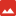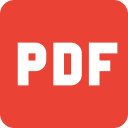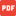Download
Phrases
Download
Sample LettersAdd.jpgBcontent.jpgLesson 01 (first two pages for sample).pdfLesson 02 (first two pages for sample).pdfLesson 03 (first two pages for sample).pdfLesson 04 (first two pages for sample).pdfLesson 05 (first page for sample).pdfLesson 06 (first page for sample).pdfLesson 07 (first page for sample).pdfLesson 08 (first page for sample).pdfLesson 09 (first page for sample).pdfLesson 10 (first page for sample).pdf